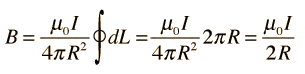# Magnetic Field Current Loop

• jromega3

## Homework Statement

A loop carries current I = 2.5 A in the x-y plane as shown in the figure above. The loop is made in the shape of a circular arc of radius R = 4 cm from qo = 60 ° to q = 360 ° . The loop is completed by horizontal and vertical sections as shown.
What is BA, the magnitude of the magnetic field at point A, the center of the circular arc?

## Homework Equations

For the loop part...## The Attempt at a Solution

Well, that's it if it were a complete loop, but this is 300/360, or 5/6 of a loop. So I take that and divide by 1.2 and I get 3.27e^-5.
Not sure about the other ones. My calculus is rusty at best.

It's hard to visualize maybe, but it's basically a circle from the 60 degree above the horizon all the way till the end, with a straight line going down to the horizontal center line and then a line connecting that line to the 0 degree mark on the curve.

As always, any help is appreciated.